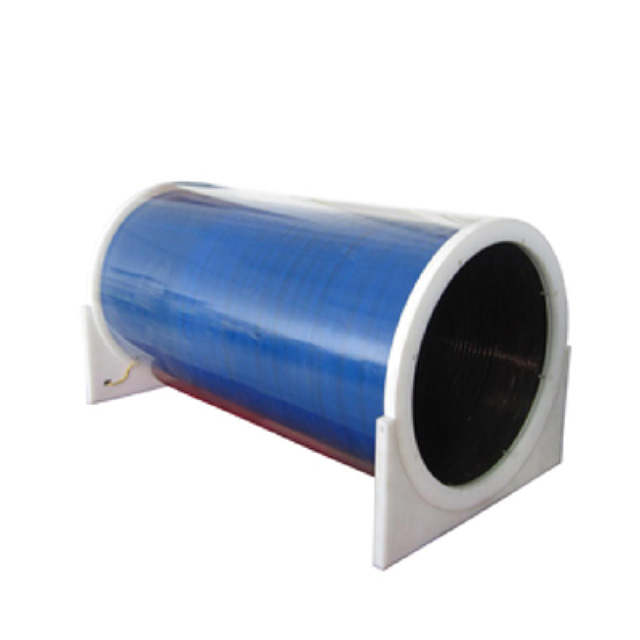#### products categories

• Tel : 18030236818
• Fax : +86-592 5237901
• Email : dexing@china-dexing.com

# Magnetic field distribution and direction of solenoid

A solenoid  is a type of electromagnet formed by a helical coil of wire whose length is substantially greater than its diameter, which generates a controlled magnetic field. The coil can produce a uniform magnetic field in a volume of space when an electric current is passed through it.

## What is a solenoid?

A solenoid is a three-dimensional coil. In physics, the term solenoid refers to a wire that is multiple coils, either hollow inside or with a metal core. When an electric current passes through the wire, a uniform magnetic field is generated inside the solenoid. Solenoids are very important components. The correct operation of many physical experiments requires a uniform magnetic field due to solenoid. Solenoids can also be used as electromagnets or inductors.

## What is the right hand rule for electrified solenoid?

Extend the right hand so that the thumb is perpendicular to the other four fingers, and all of them are in the same plane with the palm; Enter THE MAGNETIC WIRE PERPENDICULAR TO THE PALM AND POINT THE THUMB IN THE DIRECTION OF THE wire movement. The direction indicated by the four fingers is the direction of the induced current. This is the right hand rule for determining the direction of the induced current when a wire cuts a magnetic inductive line. The right hand rule determines the relationship between the current direction of the conductor cutting magnetic induction line and the conductor movement direction. The right-hand HELical rule (amperometric rule) determines the relationship between the direction of the current in the conducting wire or coil and the direction of the magnetic induction line produced by it.## What is magnetic field inside a solenoid ?

The solenoid is like a strip magnet. When it is suspended, the N pole points north and the S pole guides, which means the solenoid is stationary in the north and south direction, due to the action of the geomagnetic field.

## What is the magnetic field of a magnetic field of solenoid formula?

solenoid formula Biot-savart law: dB=(U *I*dl)/(4 * 3.14* R ^2). For ampere's law solenoid the energized solenoid and the magnetic field on its axis: dB=(U *R^2*I* N *dx)/(2(x^2+R^2)^1.5) by integration: L represents the length of the solenoid,R is the radius of the solenoid,I is the magnitude of the current, N is the number of turns, and u is 4*3.14*10^(-7) n /A^2. When R < < L, for the ideal case, the wireless long solenoid axis has B=u*n*I at any point, and B=0 outside the tube. In fact, the axis of the wireless long solenoid has B= (approximately equal to) 0.5u*n*I at any point.

## How does magnetic field flow in solenoid?

The magnetic lines inside, you can think of as parallel, so the magnetic field is the same strength. It's also the biggest. At both ends of the solenoid, the magnetic field intensity is about equal to the intensity in the tube, the magnetic induction line, radiating from both ends, so the farther away from both ends, the magnetic field intensity is about small, to infinity is zero, and magnetic field outside a solenoid, the magnetic field through a solenoid intensity in the middle of the solenoid is zero.

## Why is an MRI a solenoid?

MRI scanners use very strong radio waves and magnetic fields that can interact with protons in tissue to create a signal that can be processed to form an image of the body. The working principle of magnetic resonance is simply to say that the hydrogen atom nuclei in the tissue cells examined have resonance phenomenon under the action of strong multilayer solenoid solenoid magnetic field calculation. This phenomenon is recorded by the resonance track of the magnetic resonance instrument, and then the high-energy electronic computer in the instrument is used to reconstruct the data, thus forming the magnetic resonance image.